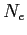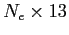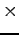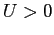Next: vertspace: input/output Up: Input/Output files Previous: celldata: input/output   Contents

### edgedata: input/output

Contains edge-centered data for each processor. (total edges) Size:• Type: ASCII
• Format: (float)Edge length
• (float)Voronoi length
• (float2)x-y components of unique normal
• (float2)x-y coordinates of center of edge
• (int)Number of edges in vertical
• (int)Maximum number of neighboring cells in vertical
• (int)Upwind cell neighbor (if)
• (int)Downwind cell neighbor (if)
• (int2) gradf pointers (see Section 3)
• (int)Edge marker

2014-08-06